Question

Circuits

1. The amplifier of Fig. 1 consists of two identical common-emitter amplifiers connected in cascade. Observe that the input resistance of the second stage, Rin2, constitutes the load resistance of the first stage.

\mathrm{mV} \text { , determine the de collector current and de collector voltage of each transistor }

(b) Draw the small-signal equivalent circuit of the entire amplifier and give the values of allits components (ignore ro).

\text { (c) Find } R_{i n} 1 \text { and } v_{b} / v_{s i g} \text { for } R_{s i g}=5 \mathrm{k} \Omega

\text { (e) For } R_{L}=2 \mathbf{k} \Omega, \text { find } v_{d} V_{b 2}

\text { (f) Find the overall voltage gain } v d v_{s i_{8}}Verified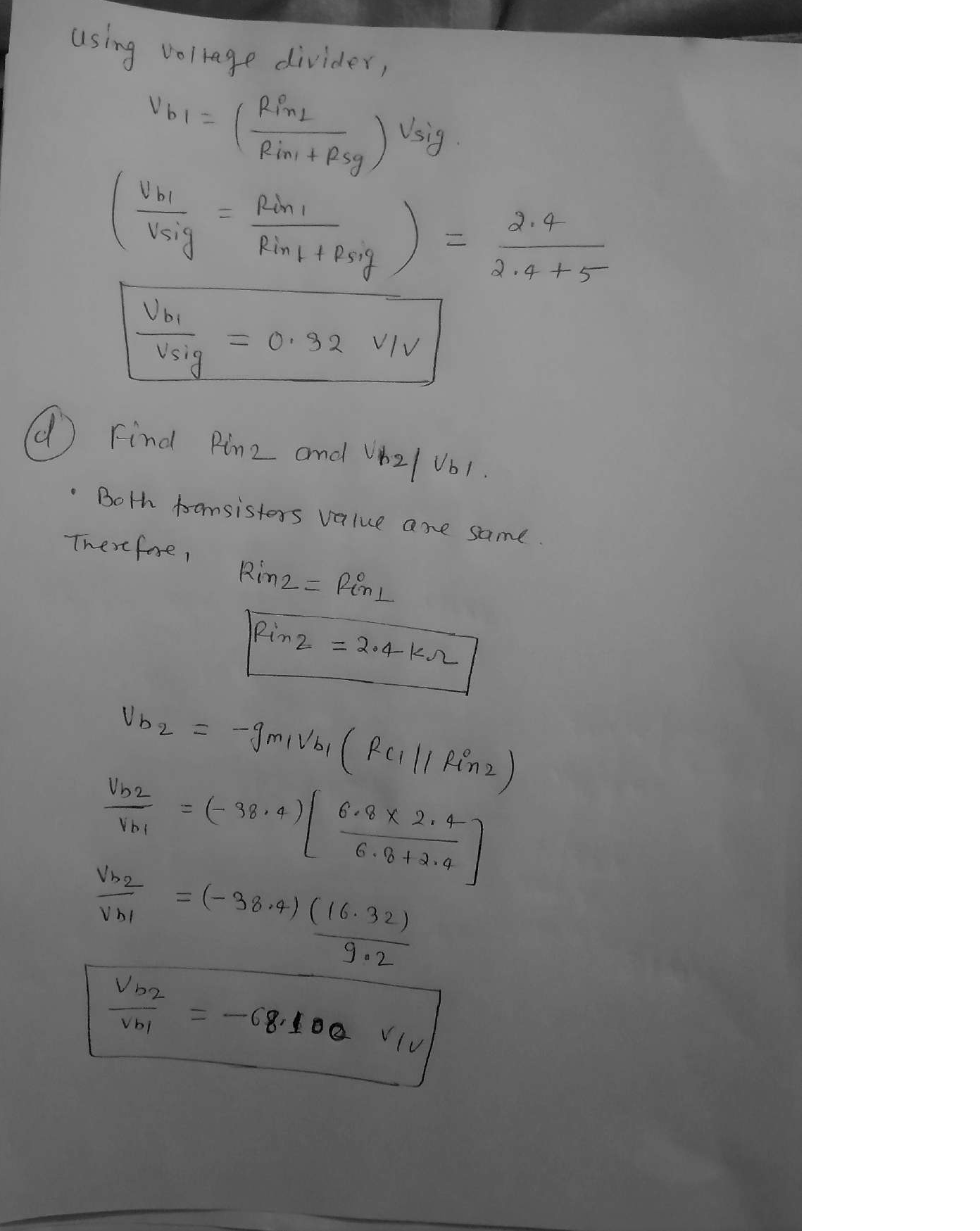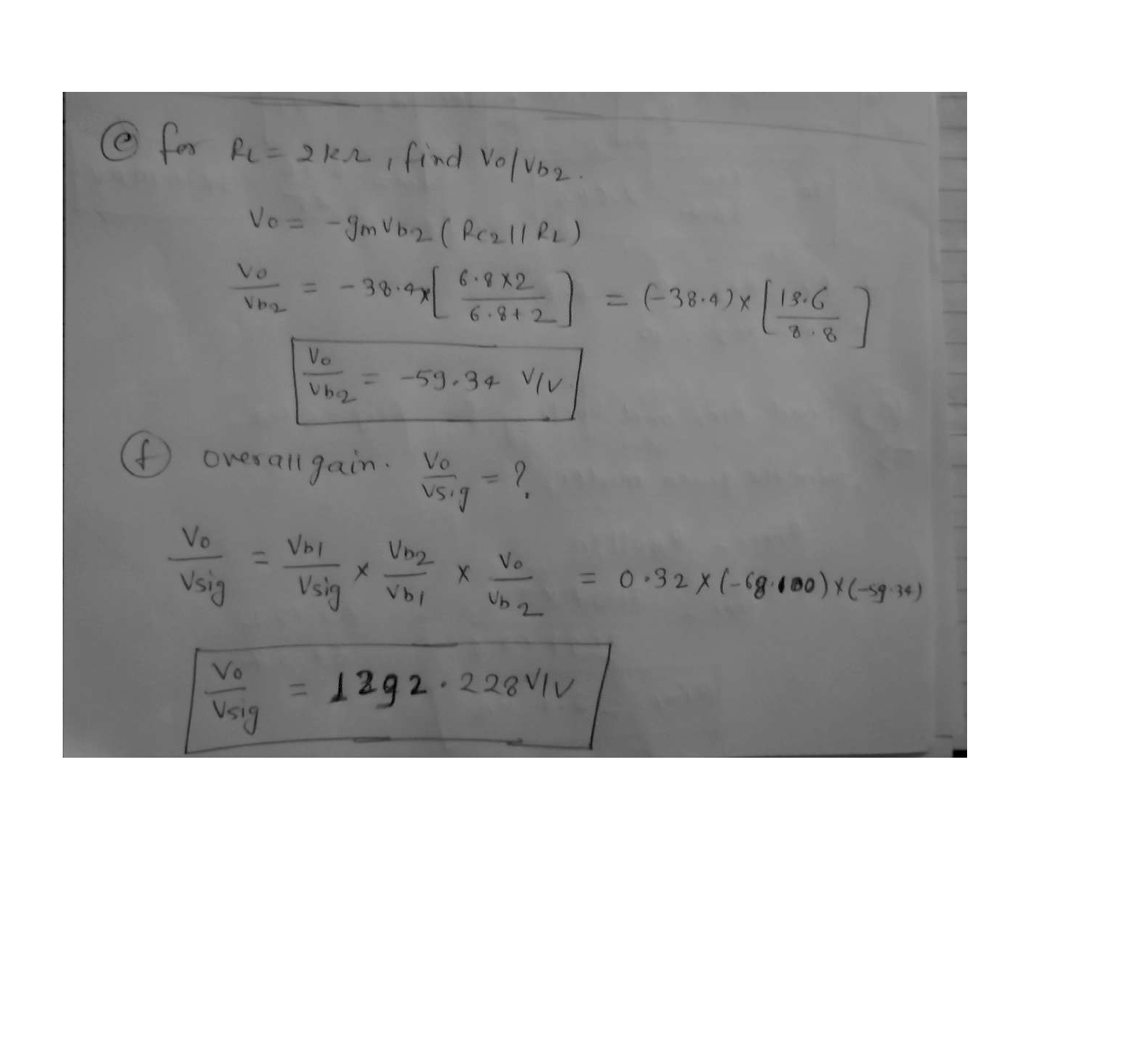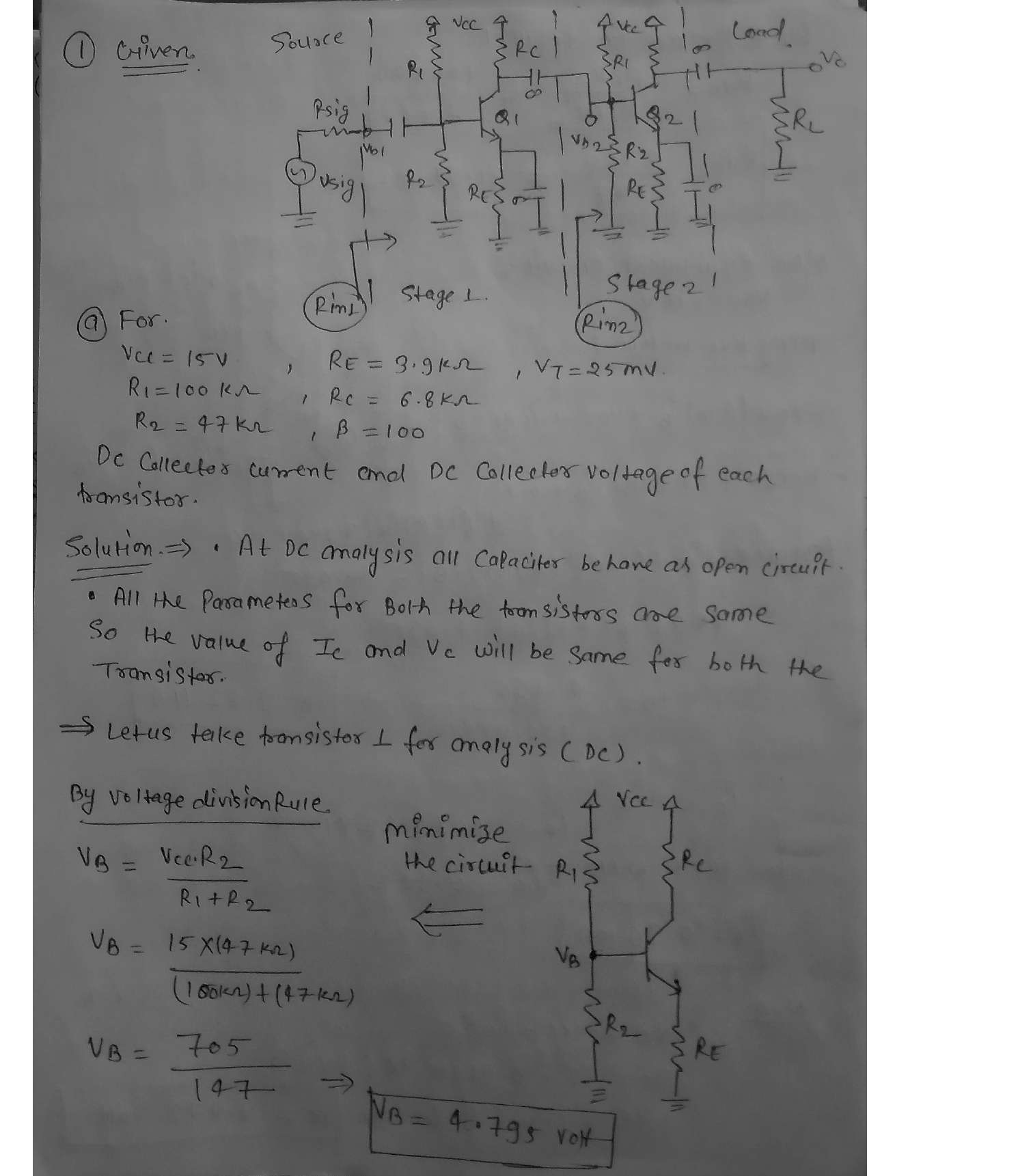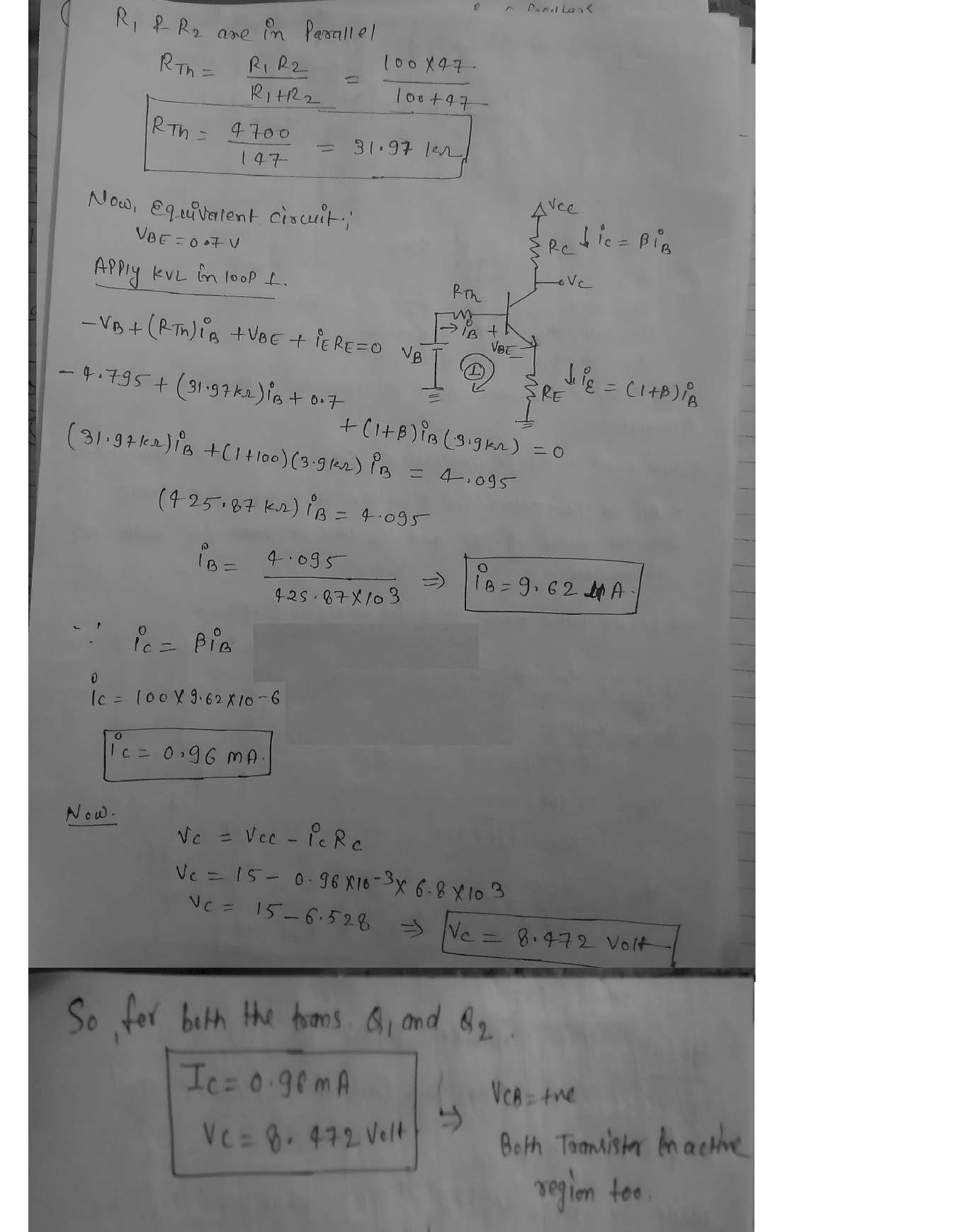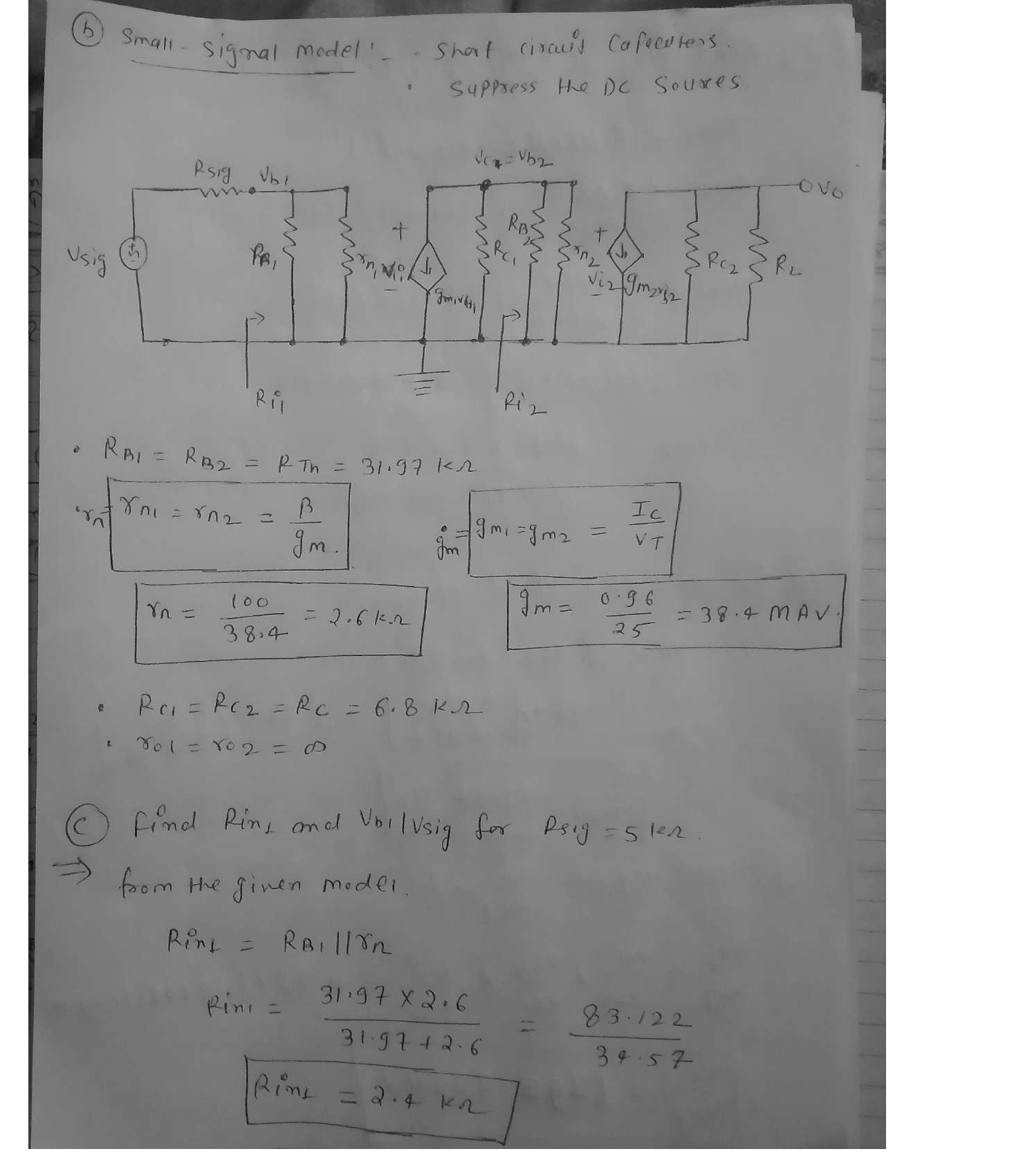### Question 52583Circuits

Problem 3 For the following circuit, use nodal analysis to write the FOUR equations in standard form. DO NOT SOLVE. You must identify any quasi-supernodes, supernodes, auxiliary equations, and remove dependent variables. Your final equations may only have V₁, V2, V3 and/or V4 as unknowns. Put your final equations in standard form in the box provided.

### Question 52582Circuits

Problem 2 For the following circuit, write the FOUR mesh equations in standard form. DO NOT SOLVE. You must identify any super meshes, auxiliary equations, and re-move all dependent variables. Your equations may only have 11,12,13 and/or 14 as unknowns. Put your final equations in standard form in the box provided.

### Question 52581Circuits

Problem 1.1 Determine Reg in Ohms at the (a,b) terminals for the following circuit. Round your answer to the nearest single digit decimal place (tenths).
Problem 1.2 Determine the voltage V, in Volts for the following circuit. Round your answer to the nearest single digit decimal place (tenths).
Problem 1.3 Determine the current I, in Amps for the following circuit. Round your answer to the nearest single digit decimal place (tenths).
Problem 1.4 Determine the power, P3n, delivered to the 302 resistor in Watts for the following circuit.Round your answer to the nearest single digit decimal place (tenths).

### Question 45529Circuits

11) From your answer in (10), does the 4V 'DC' or steady state voltage impact the value of the displacement current, Ic.

### Question 45528Circuits

\text { 10) Assuming } I_{C}=C \frac{d V_{C}}{d t} \text {, find the displacement current for } V_{C}=e^{-\omega t}+4 V \text { and } V_{C}=
sin(wt) + 4 V.

### Question 45527Circuits

9) Based on your answer in (7), can you apply an arbitrarily large field or voltage across a dielectric? What is the name for this limitation and what is its value for Air and Glass (SiO2)?

### Question 45526Circuits

8) Remembering quantum tunneling from Chapter 3, what is happening to the probability of a tunneling event as the "thickness" of the barrier representing the dielectric decreases?

### Question 45525Circuits

7) What happens to the relative "thickness", or distance an electron must travel in a straight line across the dielectric, as you increase the voltage?

### Question 45524Circuits

6) Redraw the diagram in (5) with a small positive voltage on the right-hand side (no voltage or OVon the left-hand side).

### Question 45523Circuits

5) Draw the energy band diagram of a capacitor made of two aluminum plates with a glass dielectric. The capacitor should have zero applied voltage.

### Submit query